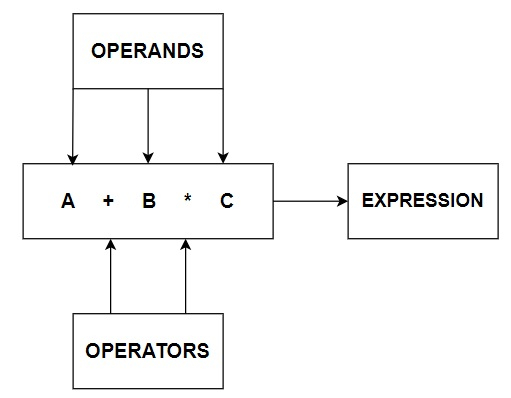# What are the logical operators in Java?

### OPERATORS

In computer programming we often need to perform some arithmetical or logical operations. In such circumstances, we need operators to perform these tasks. Thus, an Operator is basically a symbol or token, which performs arithmetical or logical operations and gives us meaningful result. The values involved in the operation are called Operands.

Here is a basic Pictorial representation of Operators.Now, let us discuss the types of Operators available.

### TYPES OF OPERATORS

There are three types of Operators in Java which are −

• Arithmetical Operators: +, -, *, -, /, %, etc.

• Relational Operators: < (Less than), >(Greater than), == (Equal to), < = (Less than or Equal to), etc.

• Logical Operators: && (AND), || (OR), ! (NOT)

## Logical OPERATORS

Java uses logical operators AND (&&), OR (||) or NOT (!). These operators yield 1 or 0 depending upon the outcome of different expressions. The different types of logical operators along with their format are as shown below −

Logical Operators Symbol Syntax
AND && (a > b) && (a > c)
OR || (a == b) || (a == c)
NOT ! !(a == b)

Precedence of logical operators is NOT (!), AND (&&) and OR (||) i.e., if a statement contains all the three logical operators then NOT operator will perform first. Here are the details of each logical operator.

### Logical NOT (!)

Logical NOT operator is applied when you want to revert the outcome of an expression. It is a unary operator because it uses a single operand.

#### Example

!(8 > 3) − False, because 8>3 is True, !(3 > 8) − True, because 3>8 is False.

public class LogicalNOT {  // public class declaration
public static void main(String[] args) {  // main function declaration
int a = 10, b = 5; // variable initialization
if (!(a == b)){  // if checking whether a is not equal to b
System.out.println("a is not equal to b"); // Printing output if a and b aren't equal
} else {
}
}
}


#### Output

a is not equal to b

### Logical OR (||)

This operator is used to combine two conditional expressions. It will result in true if either of two conditions (expressions) is true otherwise false.

#### Example

(5 > 4) || (8 > 12) : True, because 5>4 is True, (5 < 4) || (8 > 12) : False, because both the expressions are false.

public class LogicalOR {  // public class declaration
public static void main(String[] args){  // main function declaration
int a = 10, b = 5, c = 13; // variable initialization
if ((a > b) || (a > c)){  // if checking whether a is maximum element or not
System.out.println("Max Element is "+ a); // Printing output
}
else if ((b > a) || (b > c)) {  // if checking whether b is maximum element or not
System.out.println("Max Element is "+ b); // Printing output
}
else if ((c > a) || (c > b)) {  // if checking whether c is maximum element or not
System.out.println("Max Element is "+ c); // Printing output
}
}
}


#### Output

Max Element is 10


Here in the first if condition, a > b is satisfied, so the first if block is executed and the rest are skipped. That is the reason why 10 is printed as Max Element instead of 13.

### Logical AND (&&)

The AND operator results in true if both the expressions (comprising its operands) are true.

#### Example

(5 > 3) && (4 > 3) : True, because both the expressions are True, ( 5 > 3) && ( 3 > 5) : False, because 3 > 5 is False.

public class LogicalAND {  // public class declaration
public static void main(String[] args){  // main function declaration
int a = 10, b = 5, c = 13; // variable initialization
if ((a > b) && (a > c)){ // if checking whether a is maximum element or not
System.out.println("Max Element is "+ a); // Printing output
}
else if ((b > a) && (b > c)) {  // if checking whether b is maximum element or not
System.out.println("Max Element is "+ b); // Printing output
}
else if ((c > a) && (c > b)){  // if checking whether c is maximum element or not
System.out.println("Max Element is "+ c); // Printing output
}
}
}


#### Output

Max Element is 13


Note that relational operators have higher precedence over logical operators AND and OR. Hence, while using AND and OR operators you need not enclose the operands within parenthesis. NOT (!) operator has the highest precedence, so it is required to enclose the operand under parenthesis.